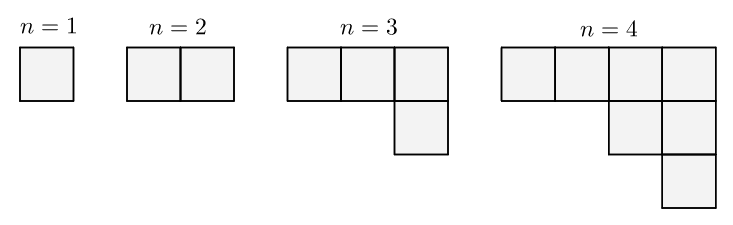# Counting Fibonacci Squares

Probability Level 1Consider the above sequence. In step $n$, we add $F_n$ (Fibonacci number) unit squares in a column to the right. We want to count the number of squares (of all sizes) in each figure.

If $n = 1$, then we count 1 square.

If $n = 2$, then 1 small square is added, so we count 2 1-by-1 squares.

If $n = 3$, then 2 small squares are added, so we count 4 1-by-1 squares.

If $n = 4$, then 3 small squares are added, so we count 7 1-by-1 squares and a 2-by-2 square, which altogether makes 8 squares in all.

For $n = 5$, is the number of all possible different-sized squares 16?

×

Problem Loading...

Note Loading...

Set Loading...